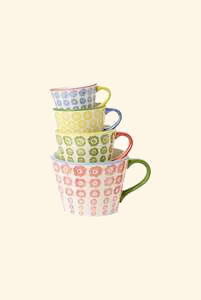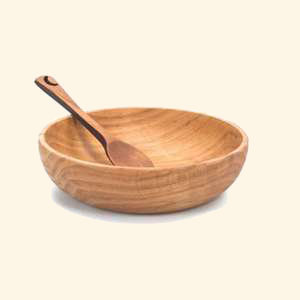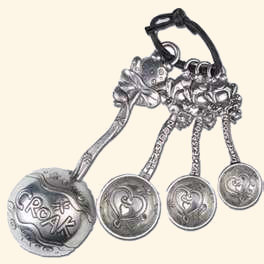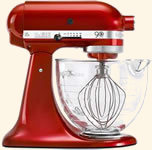Cooking Conversions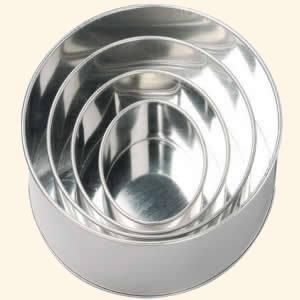Photo Credit: Squires Kitchen

# Cooking Length Conversions

Conversions of units of length are not very common in recipes, mainly being seen in cake making with the size of the tin that the mixture should fill being given in inches (in) or centimetres (cm). For instance a recipe may specify a 10" baking tin or a 20cm circular cake tin. Sometimes you will see a layer of an ingredient specified as 5mm or ¼in which will need to be converted. Below is a converter for inches to centimetres and centimetres to inches and further down the page is a converter for inches to millimetres and millimetres to inches.

## Inches to Centimetres Converter

Type a value in either field to convert from inches to centimetres (in to cm) or centimetres to inches (cm to in) (results are accurate to two decimal places).

 Inches Centimetres in = cm

## Common Inch to Centimetre Conversions Table

Most of the common inch to centimetre conversions are given in the table below (results are accurate to one decimal place).

 Inches 1 2 3 4 5 6 7 8 9 10 11 12 Centimetres 2.5 5 7.5 10 12.5 15 17.5 20 22.5 25 27.5 30

## Inch to Centimetre Conversion Formula

The conversion formula for inches to centimetres (in to cm) is 1 inch = 2.54 centimetres.

## Inches to Millimetres Converter

Type a value in either field to convert from inches to millimetres (in to mm) or millimetres to inches (mm to in) (results are accurate to one decimal place).

 Inches Millimetres in = mm

## Inch to Millimetre Conversion Formula

The conversion formula for inches to millimetres (in to mm) is 1 inch = 25.4 millimetres.